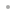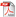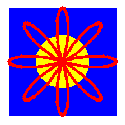GeometricaPlusContentsWhat's NewExamplesReferencesInstallation InstructionsBuy Online# GeometricaPlus

### Exact Drawing and GeometryGeometricaPlus by 3P lets users perform a wide range of geometrical operations and analysis using the list processing, symbolic programming, and functional programming facilities of the Wolfram Language. GeometricaPlus introduces interactivity in animated examples, access to libraries, and precise intersections. It maintains and deepens four approaches: Cartesian, Euclidean, parametric, and differential geometry.

Cartesian Geometry
In Cartesian or analytical geometry, a point is defined by two or three coordinates, and curves and surfaces are defined by their equations. Such functions are noted with the capital letter C at the beginning of the function to avoid confusion, such as CPoint, CLine, CConic, and CPlane to denote a Cartesian point, line, conic, and plane.

Mathematica regions also use Cartesian geometry and associate a polynomial with explicit variables such as x, y, or z to a geometrical object. In GeometricaPlus, the variables are absent, and the coefficients of the polynomial suffice to define the object. In other terms, the concept of coordinates is generic from points to quadrics. Last, it is to be noted that the Cartesian definition is unique, but does not always exist, hence the interest of parametric geometry.

Euclidean Geometry
In Euclidean or synthetic geometry, the point is a primitive, and there is a variety of ways of defining geometrical objects. This great variety is very helpful in practical applications and is unified by theorems. Many functions specifically related to Euclidean geometry start with a capital E, such as ELine and ECircle.

Parametric Geometry
Parametric definitions are well adapted for the representation of geometrical objects and for elements of objects such as bound points, segments, arcs, or limited surfaces. It is, however, not unique. The parametric representation chosen in GeometricaPlus is given by the function Pointer. The capital P in PPoint or PRange denotes the parametric definition. A non-Cartesian curve or surface is an object of head PPoint depending on one or two parameters in intervals defined by the option PRange.

Differential Geometry
Whereas Cartesian or Euclidean geometries deal with global objects, differential geometry applies to the local properties of Cartesian or, more generally, parametric objects. Curvature, torsion, evolutes, isolines, and generalized parallelism are examples of properties that can be determined using GeometricaPlus.

GeometricaPlus is used by mathematics educators at the high school and college level, by researchers for drawing exact diagrams of any complexity, and by graphical designers, architects, and engineers for CAD applications.

Developed and supported by 3P (Les Trois Platanes).

Les Trois Platanes
Les Clots
26770 Montbrison-sur-Lez
France
+33-(0)6-87-02-63-55
contact@troisplatanes.com

GeometricaPlus requires Mathematica 10 and is available for Windows, Mac OS X, and Linux.